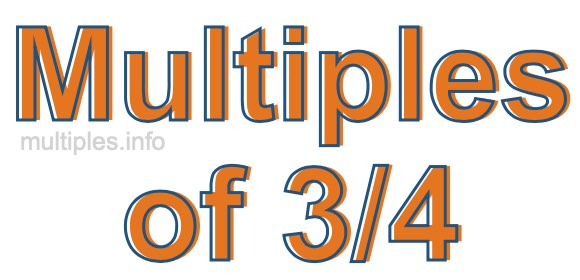Multiples of 3/4We define the multiples of 3/4 as the product of any integer multiplied by 3/4. Therefore, to create a list of multiples of 3/4 we start by multiplying 3/4 by one, then we multiply 3/4 by two, then we multiply 3/4 by three, and so on.

To multiply a fraction by an integer, we leave the denominator alone and multiply the numerator by the integer. It is impossible to give you a list of all multiples of 3/4 because the list goes on forever. However, here is the math to calculate the beginning list of multiples of 3/4.

3/4 × 1 = 3/4

3/4 × 2 = 6/4

3/4 × 4 = 9/4

3/4 × 4 = 12/4

3/4 × 5 = 15/4

3/4 × 6 = 18/4

3/4 × 7 = 21/4

3/4 × 8 = 24/4

3/4 × 9 = 27/4

3/4 × 10 = 30/4

3/4 × 11 = 33/4

3/4 × 12 = 36/4

We have simplified the fractions above for you. Below are the multiples of 3/4 in their lowest possible terms:

3/4 = 3/4

6/4 = 1 1/2

9/4 = 2 1/4

12/4 = 3

15/4 = 3 3/4

18/4 = 4 1/2

21/4 = 5 1/4

24/4 = 6

27/4 = 6 3/4

30/4 = 7 1/2

33/4 = 8 1/4

36/4 = 9

As we stated above, the list of multiples of 3/4 goes on forever because the product of 3/4 multiplied by any whole number (integer) is a multiple of 3/4. You can also create a list of multiples of 3/4 if you keep adding 3/4 like this:

3/4
3/4 + 3/4 = 6/4
3/4 + 3/4 + 3/4 = 9/4
3/4 + 3/4 + 3/4 + 3/4 = 12/4

Again, the list can go on forever, because you can continue adding 3/4 to the list. Therefore, the list of multiples of 3/4 is ∞ (infinite).

Multiples of Fractions
Do you need the multiples of another fraction? Enter a another fraction below to get the multiples of that fraction.

/

Multiples of 3/5
Go here for the next fraction on our list that we have multiples for.